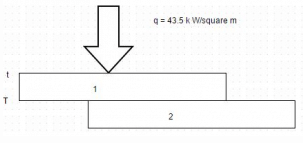Test: General Heat Conduction Equation

# Test: General Heat Conduction Equation

Test Description

## 10 Questions MCQ Test Heat Transfer | Test: General Heat Conduction Equation

Test: General Heat Conduction Equation for Chemical Engineering 2022 is part of Heat Transfer preparation. The Test: General Heat Conduction Equation questions and answers have been prepared according to the Chemical Engineering exam syllabus.The Test: General Heat Conduction Equation MCQs are made for Chemical Engineering 2022 Exam. Find important definitions, questions, notes, meanings, examples, exercises, MCQs and online tests for Test: General Heat Conduction Equation below.
Solutions of Test: General Heat Conduction Equation questions in English are available as part of our Heat Transfer for Chemical Engineering & Test: General Heat Conduction Equation solutions in Hindi for Heat Transfer course. Download more important topics, notes, lectures and mock test series for Chemical Engineering Exam by signing up for free. Attempt Test: General Heat Conduction Equation | 10 questions in 20 minutes | Mock test for Chemical Engineering preparation | Free important questions MCQ to study Heat Transfer for Chemical Engineering Exam | Download free PDF with solutions
 1 Crore+ students have signed up on EduRev. Have you?
Test: General Heat Conduction Equation - Question 1

### From the concept of kinetic theory, mean travel velocity of the gas molecules is prescribed by the relation Where, G = Universal gas constant M = Molecular weight of the gas T = Absolute temperature

Detailed Solution for Test: General Heat Conduction Equation - Question 1

Heat transfer by conduction in gases occurs through transport of the kinetic energy of molecular motion resulting from the random movement and collisions of the molecules.

Test: General Heat Conduction Equation - Question 2

### Low temperature insulation are used when the enclosure is at a temperature lower than the ambient temperature. Which one of the following is not a low temperature insulation?

Detailed Solution for Test: General Heat Conduction Equation - Question 2

Asbestos is a high temperature insulation which are used when it is desired to prevent an enclosure at a temperature higher than the ambient from losing heat to its surroundings.

Test: General Heat Conduction Equation - Question 3

### The value of Lorenz number in 10-8 W ohms/K2 is

Detailed Solution for Test: General Heat Conduction Equation - Question 3

Lorenz no. = k/α T

Test: General Heat Conduction Equation - Question 4

For liquids, thermal conductivity is governed by the relation

Where,
C= Specific heat at constant pressure
M = Molecular weight of the liquid
p = Mass density
A = Area

Detailed Solution for Test: General Heat Conduction Equation - Question 4

Area doesn’t depends on the nature of liquid but on the quantity (Ac p) is nearly constant for all liquids.

Test: General Heat Conduction Equation - Question 5

Consider the following parameters
(i) Composition
(ii) Density
(iii) Porosity
(iv) Structure
Then, thermal conductivity of glass wool varies from sample to sample because of variation is

Detailed Solution for Test: General Heat Conduction Equation - Question 5

There is a variation due to all above options.

Test: General Heat Conduction Equation - Question 6

The thermal conductivity and the electrical conductivity of a metal at absolute temperature are related as

Detailed Solution for Test: General Heat Conduction Equation - Question 6

It is defined as the ratio of thermal conductivity to the product of electrical conductivity and temperature.

Test: General Heat Conduction Equation - Question 7

The relation Ϫ2 t =0 is referred to as

Detailed Solution for Test: General Heat Conduction Equation - Question 7

In the absence of internal heat generation or release of energy within the body, equation reduces to Laplace equation.

Test: General Heat Conduction Equation - Question 8

The unit of thermal diffusivity is

Detailed Solution for Test: General Heat Conduction Equation - Question 8

The quantity α = k/pc is called thermal diffusivity.

Test: General Heat Conduction Equation - Question 9

To effect a bond between two metal plates, 2.5 cm and 15 cm thick, heat is uniformly applied through the thinner plate by a radiant heat source. The bonding must be held at 320 K for a short time. When the heat source is adjusted to have a steady value of 43.5 k W/m2, a thermocouple installed on the side of the thinner plate next to source indicates a temperature of 345 K. Calculate the temperature gradient for heat conduction through thinner plate. In the diagram, the upper plate is 2.5 cm thick while the lower is 15 cm thick.Detailed Solution for Test: General Heat Conduction Equation - Question 9Temperature gradient = d t/d x = – 1000 degree Celsius/m. Also, q/A = – k (t2– t 1)/δ.

Test: General Heat Conduction Equation - Question 10

The diffusion equations
Ɏ2t + q = (1/α) (d t/d r)
Governs the temperature distribution under unsteady heat flow through a homogenous and isotropic material. The Fourier equation follows from this expression when

Detailed Solution for Test: General Heat Conduction Equation - Question 10

In unsteady state condition, there is no internal heat generation.

## Heat Transfer

58 videos|70 docs|85 tests
 Use Code STAYHOME200 and get INR 200 additional OFF Use Coupon Code
Information about Test: General Heat Conduction Equation Page
In this test you can find the Exam questions for Test: General Heat Conduction Equation solved & explained in the simplest way possible. Besides giving Questions and answers for Test: General Heat Conduction Equation, EduRev gives you an ample number of Online tests for practice

## Heat Transfer

58 videos|70 docs|85 tests